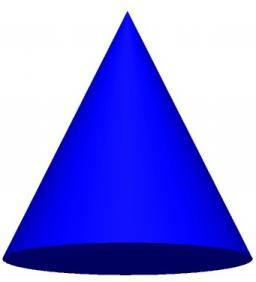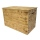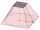# Cone

Calculate volume and surface area of ​​the cone with a diameter of the base d = 15 cm and side of cone with the base has angle 52°.

Correct result:

V =  565.5 cm3
S =  463.7 cm2

#### Solution:

$D=15 \ \text{cm} \ \\ r=D/2=15/2=\dfrac{ 15 }{ 2 }=7.5 \ \text{cm} \ \\ S_{1}=\pi \cdot \ r^2=3.1416 \cdot \ 7.5^2 \doteq 176.7146 \ \text{cm}^2 \ \\ A=52 \ ^\circ \ \\ h=r \cdot \ \tan A ^\circ =r \cdot \ \tan 52^\circ \ =7.5 \cdot \ \tan 52^\circ \ =7.5 \cdot \ 1.279942=9.59956 \ \\ V=\dfrac{ 1 }{ 3 } \cdot \ S_{1} \cdot \ h=\dfrac{ 1 }{ 3 } \cdot \ 176.7146 \cdot \ 9.5996=565.5 \ \text{cm}^3$
$s=\sqrt{ h^2 + r^2 }=\sqrt{ 9.5996^2 + 7.5^2 } \doteq 12.182 \ \text{cm} \ \\ S=S_{1} + \pi \cdot \ r \cdot \ s=176.7146 + 3.1416 \cdot \ 7.5 \cdot \ 12.182=463.7 \ \text{cm}^2$Our examples were largely sent or created by pupils and students themselves. Therefore, we would be pleased if you could send us any errors you found, spelling mistakes, or rephasing the example. Thank you!

Leave us a comment of this math problem and its solution (i.e. if it is still somewhat unclear...):Be the first to comment!Tips to related online calculators
Pythagorean theorem is the base for the right triangle calculator.

#### You need to know the following knowledge to solve this word math problem:

We encourage you to watch this tutorial video on this math problem:

## Next similar math problems:

• Cuboid to cubeA cuboid with dimensions of 9 cm, 6 cm, and 4 cm has the same volume as a cube. Calculate the surface of this cube.The surface of the regular quadrilateral prism is 8800 cm2, the base edge is 20 cm long. Calculate the volume of the prism
• Cube surfce2volumeCalculate the volume of the cube if its surface is 150 cm2.
• Cuboid edgesCalculate the volume and surface of a cuboid whose edge lengths are in the ratio 2: 3: 4 and the longest edge measures 10cm.
• The cubeThe cube has a surface area of 216 dm2. Calculate: a) the content of one wall, b) edge length, c) cube volume.
• Regular hexagonal prismCalculate the volume of a regular hexagonal prism whose body diagonals are 24cm and 25cm long.
• Copper Cu wireCopper wire with a diameter of 1 mm and a weight of 350 g is wound on a spool. Calculate its length if the copper density is p = 8.9 g/cm cubic.
• Spherical segmentCalculate the volume of a spherical segment 18 cm high. The diameter of the lower base is 80 cm, the upper base 60 cm.
• EightEight small Christmas balls with a radius of 1 cm have the same volume as one large Christmas ball. What has a bigger surface: eight small balls, or one big ball?
• The water tankThe water tank has the shape of a sphere with a radius of 2 m. How many liters of water will fit in the tank? How many kilograms of paint do we need to paint the tank, if we paint with 1 kg of paint 10 m2?
• Surface and volumeFind the surface and volume of a cuboid whose dimensions are 1 m, 50 cm, and 6 dm.
• Magnified cubeIf the lengths of the edges of the cube are extended by 5 cm, its volume will increase by 485 cm3. Determine the surface of both the original and the magnified cube.
• Wooden boxThe block-shaped box was placed on the ground, leaving a rectangular print with dimensions of 3 m and 2 m. When flipped over to another wall, a print with dimensions of 0.5 m and 3 m remained in the sand. What is the volume of the wooden box?
• AL wireWhat is the weight of an aluminum wire 250 m long with a diameter of 2 mm, if the density of aluminum is p = 2700 kg/m cubic. Determine to the nearest gram.
• Runcated pyramid teapotThe 35 cm high teapot has the shape of a truncated pyramid with the length of the edge of the lower square base a=50 cm and with the edges of the rectangular base b: 20 cm and c: 30 cm. How many liters of water will fit in the teapot?
• Concrete hatchThe concrete hatch for a round well has a diameter of 1300 mm and a thickness of 80 mm. Determine its weight in kg if the density of the concrete is 2545 kg/m3
• Aquarium heightHow high does the water in the aquarium reach, if there are 36 liters of water in it? The length of the aquarium is 60 cm and the width is 4 dm.